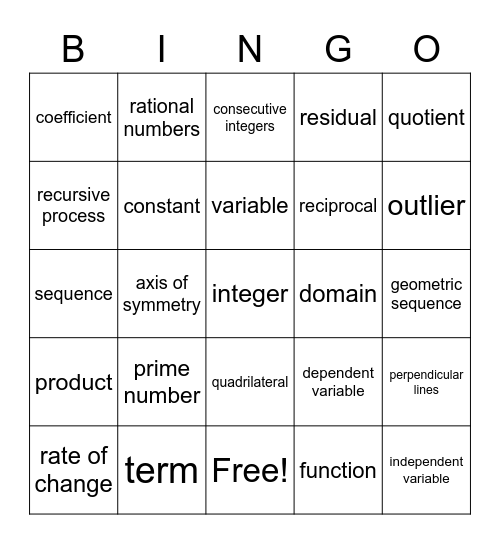# Math Vocabulary BingoThis bingo card has a free space and 31 words: Free!, arithmetic sequence, axis of symmetry, binomial, coefficient, consecutive integers, constant, dependent variable, domain, function, geometric sequence, independent variable, irrational numbers, monomial, outlier, parallel lines, perpendicular lines, prime number, product, quadrilateral, quotient, range, rate of change, rational numbers, reciprocal, recursive process, residual, sequence, term, variable and vertex.

## Play Online